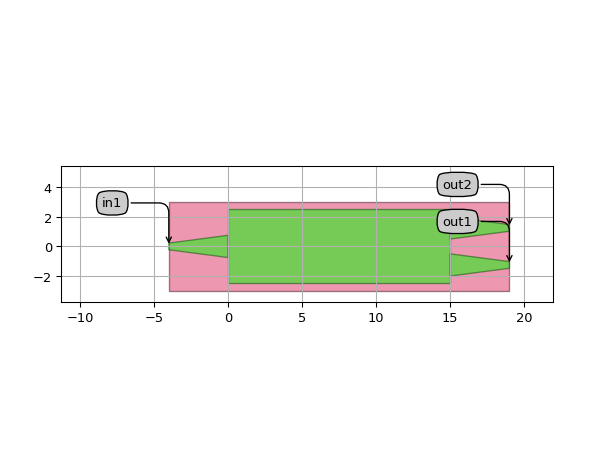# Multimode interferometer¶

SiFab contains two multimode interferometers (MMI):

• MMI1x2: a fully parametric MMI, with one input and two outputs.
• MMI1x2Optimized: an optimized 1x2 MMI with locked properties.

The section Simulation and regeneration of the data files explains how to simulate and regenerate the simulation data of an MMI. The section Creating a new optimized MMI explains how to create a new optimized MMI with different layout parameters.

## MMI1x2¶

This is an MMI with fully customisable layout. This class has a circuit model where the following parameters can be provided to perform a circuit simulation of the MMI: center_wavelength, transmission, reflection_in and reflection_out.

Reference

Click on the name of the component below to see the complete PCell reference.

 si_fab.all.MMI1x2 MMI with 1 input and 2 outputs.

Example

from si_fab import all as pdk

mmi = pdk.MMI1x2(trace_template=pdk.SiWireWaveguideTemplate(),
width=5.0,
length=15.0,
taper_width=1.5,
taper_length=4.0,
waveguide_spacing=2.5)
mmi_lv = mmi.Layout()
mmi_lv.visualize(annotate=True)### MMI Model¶

For the 1x2 MMI, we use the following model:

$\begin{split}\begin{array}{c} S[in_1, out_1] &=& S[out_1, in_1] &=& t \\ S[in_1, out_2] &=& S[out_2, in_1] &=& t \\ S[in_1, in_1] &=& r_1 &=& r_1 \\ S[out_1, out_1] &=& S[out_2, out_2] &=& r_1 &=& r_2 \end{array}\end{split}$

where $$t$$ is the transmission (through), $$r_1$$ is the reflection at the input and $$r_2$$ is the reflection at the output. $$t$$, $$r_1$$, $$r_2$$ are dispersive with respect to wavelength.

## MMI1x2Optimized¶

This MMI inherits from MMI1x2. All the properties are locked, because the layout has been optimized to obtain maximum transmission at 1550 nm wavelength. The optimization and simulation of this component have been performed using CAMFR, the built-in 2D solver of IPKISS. After the simulation, a fitting is performed of the transmission and reflections as a function of wavelength. The fitting coefficients for $$t$$, $$r1$$, $$r2$$ are stored and used by Caphe to calculate the S-matrix and perform a circuit simulation.

Reference

Click on the name of the component below to see the complete PCell reference.

 si_fab.all.MMI1x2Optimized MMI1x2 with layout parameters optimized for maximum transmission at 1550 nm.

Example

from si_fab import all as pdk
import numpy as np
import pylab as plt

mmi = pdk.MMI1x2Optimized()
mmi_lv = mmi.Layout()
mmi_lv.visualize(annotate=True)

mmi_cm = mmi.CircuitModel()

wavelengths = np.linspace(1.5, 1.6, 51)
S = mmi_cm.get_smatrix(wavelengths=wavelengths)
plt.figure()
plt.title("MMI transmission")
plt.plot(wavelengths, 10 * np.log10(np.abs(S["out1", "in1"]) ** 2), '-', linewidth=2.2, label="T(out1)")
plt.plot(wavelengths, 10 * np.log10(np.abs(S["out2", "in1"]) ** 2), '-', linewidth=2.2, label="T(out2)")
plt.plot(wavelengths, 10 * np.log10(np.abs(S["in1", "in1"]) ** 2), '-', linewidth=2.2, label="R(in1)")
plt.ylim(-60, 5)
plt.xlabel('Wavelength [um]', fontsize=16)
plt.ylabel('Transmission [dB]', fontsize=16)
plt.legend(fontsize=14, loc=5)
plt.show()## Simulation and regeneration of the data files¶

The simulation data of MMI1x2Optimized obtained with CAMFR can be regenerated. This can be done with the regenerate_mmi() function using the script regenerate_mmi_1x2_optimized.py contained in si_fab/components/mmi/regeneration. The user can specify the following options:

• mmi_class: the mmi to regenerate. The name should not contain parentheses ().
• wavelengths: list of wavelengths at which the simulation should be run.
• center_wavelength: the center wavelength of the component.
• resimulate: boolean. If True, the MMI will be simulated at all the wavelengths. A fitting of the transmission as a function of wavelength is performed and the results are stored in a file in data/component_name.z.
• plot: boolean. If True, it plots the results of the simulation.

Reference

Click on the name of the functions below to see the complete API reference of the simulation and regeneration recipes.

 si_fab.components.mmi.simulation.simulate_camfr.simulate_splitter_by_camfr It simulates a symmetric splitter and returns the transmission and reflection. si_fab.components.mmi.regeneration.regen_utils.regenerate_mmi It regenerates the simulation fitting data and/or the plots of the optimized MMI.

## Creating a new optimized MMI¶

The user has the possibility of exploring the MMI and creating a new optimized component with different optimized parameters. The optimization can be performed by running the script optimize_mmi_1x2.py contained in si_fab/components/mmi/optimization. Some layout parameters are kept fixed (taper_width, taper_length, width), while the length of the MMI (length) and the spacing between the output waveguides (wg_spacing) are optimized to obtain maximum transmission. The new layout parameters can be used to create an optimized MMI with new default values. The simulation data of this new component can be generated by running the regeneration script and should be used to perform circuit simulations of this new component.

Reference

Click on the name of the function below to see the complete API reference of the optimization recipe.

 si_fab.components.mmi.optimization.opt_utils.optimize_mmi Optimizes the length and waveguide spacing of a 1x2 MMI at fixed wavelength, taper_length and width.